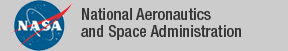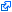### Formalization of Sturm's Theorem

Sturm's Theorem is a well-known result in real algebraic geometry that provides a function that computes the number of roots of a univariate polynomial in a semi-open interval. The PVScontribution `Sturm`, which is part of the NASA PVS Library, includes a formalization of Sturm's Theorem, as well as a decision procedure that determines the sign of a univariate polynomial on a given interval. The decision procedure uses a combination of Sturm's Theorem, an interval bisection procedure, and the fact that a polynomial with exactly one root in a finite interval is always nonnegative on that interval if and only if it is nonnegative at both endpoints. It is formally verified in PVS that the decision procedure is sound and complete. This result is a the basis of the proof-producing strategies `sturm` and `mono-poly` for reasoning about polynomial relations over a real interval. The soundness of the strategies depends solely on the internal logic of PVS rather than on an external oracle.

### PVS Contribution

• `Sturm`: Formalization of Sturm's theorem and PVS strategies (see dependency graph).
• Strategy `sturm`: Automatically discharges polynomial relations over a real interval.
• Strategy `mono-poly`: Automaically discharges monocity properties of polynomials on a real interval.
• Examples.
• Tarski's Theorem.
• ### Publications

The tagidentifies links that are outside the NASA domain